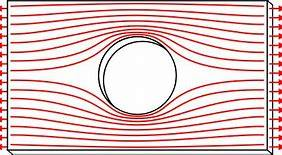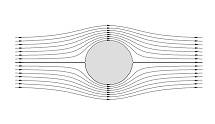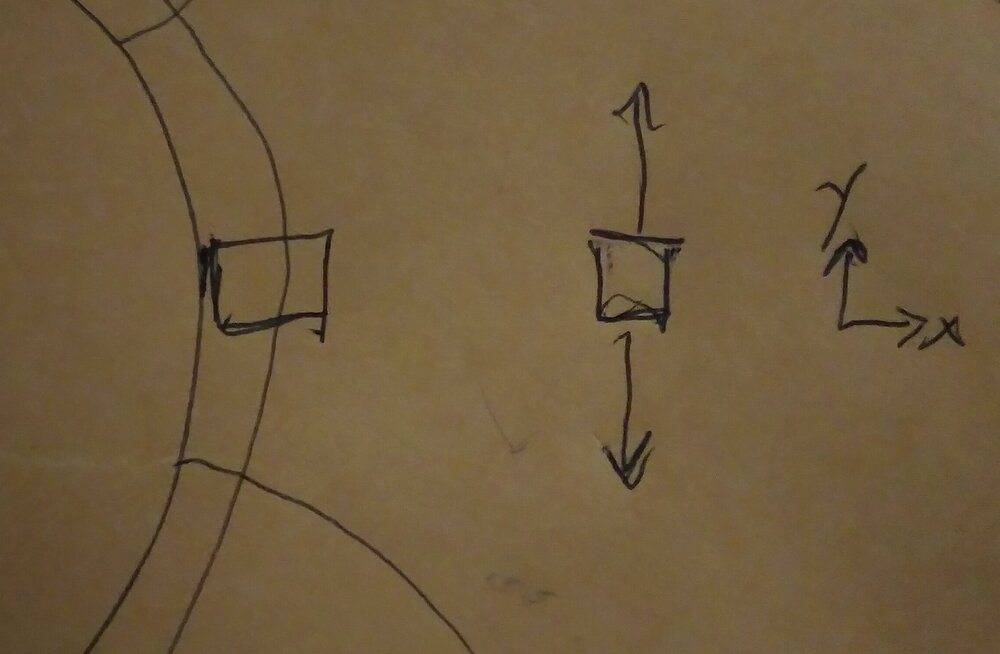# Lines of Stress in a Material

person123
TL;DR Summary
I want to check my understanding of what the lines of stress shown in a material means; I'm also curious if there's a relation between them and scalar fields which satisfy Laplace's equation, like streamlines for a fluid.
I was initially curious by the fact that streamlines around a circle appear the same as the lines of stress around a hole:I understand that streamlines are the contour lines of the stream function ##\psi## which satisfies the Laplace equation. I was wondering there is a related function for the lines of stress.

I want to make sure I understand what the lines of stress in a solid material (first image) represent. This is my understanding:

I think there are actually two orthogonal lines of stress in a material. I'm guessing that at any point in the material, the orientation of the lines are the orientation of the principal stresses. I believe that the closer the lines are together the greater the stress or ##\frac{\partial \phi}{\partial x}=\sigma_x##. However, I am not sure where to go from here to show whether the function satisfies Laplace's equation.

Last edited:

Mentor
You have shown the streamlines for inviscid flow. For inviscid flow, the stress tensor is isotropic. So there are no principal directions of stress.

person123
You have shown the streamlines for inviscid flow. For inviscid flow, the stress tensor is isotropic. So there are no principal directions of stress.
I was just showing the streamlines to show that I was curious about them appearing similar. The last paragraph was just for the lines of stress in a solid material.

Mentor
I was just showing the streamlines to show that I was curious about them appearing similar. The last paragraph was just for the lines of stress in a solid material.
Like I said, the stress tensor is isotropic in inviscid flow.

person123
Like I said, the stress tensor is isotropic in inviscid flow.
My question is really just what exactly the lines of stress in a solid material represent and whether they are contours of functions which satisfy Laplace's equation. The part about streamlines is just to show why I suspect that might be the case. I'm only suggesting that they are mathematically similar, while what they represent is physically completely different. I think I could also relate it to lines of equal temperature with a circular heat source or equipotential lines for a circular charged object.

Mentor
My question is really just what exactly the lines of stress in a solid material represent and whether they are contours of functions which satisfy Laplace's equation. The part about streamlines is just to show why I suspect that might be the case. I'm only suggesting that they are mathematically similar, while what they represent is physically completely different. I think I could also relate it to lines of equal temperature with a circular heat source or equipotential lines for a circular charged object.
I strongly doubt that the principle directions of stress are aligned as you have suggested in a solid. Certainly, in a solid, the stresses on surfaces normal to the cylinder would have a shear component.

person123
Wouldn't there be no shear stress on surface normal to the cylinder? This is what I would imagine the internal stress adjacent to the hole would be:Because the surface tangential to the hole can't exert any stress ##\sigma_x=0## and ##\tau_{xy}=0##. This would mean there's uniaxial tension. This would also mean the shear stress normal to the cylinder would also be 0, but shear stresses at an angle would be nonzero.

According to https://www.oxfordreference.com/view/10.1093/oi/authority.20110803100537146, stress trajectories (which I think is what I meant when I was saying lines of stress) are in the orientation of principal stresses. Maybe it is referring to something else though.

Last edited:
Mentor
Wouldn't there be no shear stress on surface normal to the cylinder? This is what I would imagine the internal stress adjacent to the hole would be:
View attachment 263627
Because the surface tangential to the hole can't exert any stress ##\sigma_x=0## and ##\tau_{xy}=0##. This would mean there's uniaxial tension. This would also mean the shear stress normal to the cylinder would also be 0, but shear stresses at an angle would be nonzero.

According to https://www.oxfordreference.com/view/10.1093/oi/authority.20110803100537146, stress trajectories (which I think is what I meant when I was saying lines of stress) are in the orientation of principal stresses. Maybe it is referring to something else though.
Who said anything about a "hole?" A hole is not a solid cylinder.

The deformation of a solid is much more like the flow of a viscous fluid in the limit of negligible inertial effects (slow flow) than that of an inviscid fluid. And certainly there is a major difference in behavior between viscous fluids and inviscid fluids in this limit.

Mentor
For inviscid flow, your hypothesis is bogus because the stress tensor, as I said, is isotropic. It doesn't even work for the simplest viscous flow of shear between two infinite parallel plates, where the principal directions of stress are at 45 degrees to the streamlines. And, for the simple case of a Hookean solid being sheared between two infinite parallel plates, the principal stresses are also at 45 degrees to the direction of shear. So, in short, your hypothesis is totally bogus.

person123
Who said anything about a "hole?" A hole is not a solid cylinder.
I was initially curious by the fact that streamlines around a circle appear the same as the lines of stress around a hole:

My question is about stress trajectories (what I was calling lines of stress because I didn't know the correct term). Here is what I'm wondering about it:
1. What is the orientation of stress trajectories (a google search makes me believe it's in the orientation of principal stresses)?
2. Could one define a scalar field whose contour lines are the stress trajectories? Would this be at all meaningful?
3. What do stress trajectories being closer together, or the gradient of the proposed field, mean? I imagine it's proportional to stress, but I don't know how to prove this.
4. Does the proposed scalar function satisfy Laplace's equation?
I was comparing stress trajectories of a solid material with a circular hole to streamlines of flow around a solid circle. I was merely suggesting that they're mathematically equivalent, while they physically represent completely different things. I was not looking at the stress within an inviscid fluid at all.

Mentor
My question is about stress trajectories (what I was calling lines of stress because I didn't know the correct term). Here is what I'm wondering about it:
1. What is the orientation of stress trajectories (a google search makes me believe it's in the orientation of principal stresses)?
I don't know what their interpretation means. Yours is fine.
1. Could one define a scalar field whose contour lines are the stress trajectories? Would this be at all meaningful?

No. In the very simple shear deformation I described, the directions of the principal stresses are the same at all point within the flow field. So there is no scalar or gradient of a scalar that would be consistent with this.

1. What do stress trajectories being closer together, or the gradient of the proposed field, mean? I imagine it's proportional to stress, but I don't know how to prove this.
:For the simplest of flow I described, there is no such thing as the principal directions of stress being further apart or closer together. The are identical at all locations in the flow field.
1. Does the proposed scalar function satisfy Laplace's equation?
There is no such scalar function.[/quote]

person123
No. In the very simple shear deformation I described, the directions of the principal stresses are the same at all point within the flow field. So there is no scalar or gradient of a scalar that would be consistent with this.
Wouldn't that be consistent with a scalar field? The stress trajectories would be equally spaced parallel lines at a 45 degree angle from the plates. These equally spaced lines would be the contours of a plane.

To clarify this is entirely in reference to the stress in a solid, not a fluid.

Last edited:
Mentor
Wouldn't that be consistent with a scalar field? The stress trajectories would be equally spaced parallel lines at a 45 degree angle from the plates. These equally spaced lines would be the contours of a plane.

person123
I'm going to try to clarify what I'm asking one more time and if it doesn't make sense or you already answered I'll stop.

Disregard the discussion of the fluid entirely. This is entirely about the discussion of the stresses in a solid.

There are stress trajectories describing the state of stress for that solid material. Are those stress trajectories contour lines of a scalar field?

Gold Member
This may or may not help but it is a way to visualize the actual stress lines in a shape. I posted this in another thread a few days ago, hope it helps.
Another way to evaluate strain concentration is to use a transparent or translucent plastic to make test versions of your holder. Illuminate it with, or shine polarized light thru it and view it thru another polarizer acting as the analyzer.

You will see color bands that show where the strain concentrations are. The closer the bands, the higher the strain at that area. You can take photos thru the second polarizer for documentation or easier comparison later.

An easy source of polarizers is Polarized sunglasses. Cut/break them apart and use one lens for the source polarizer and the other for viewing. You will have to rotate one or both of them to get the best effect.

Note: Not all Polarized sunglasses really are. There are some knockoffs on the market. Test by shining a light thru, or looking at a light thru, two of them and rotate one of them. Transmission should vary between
maximum and minimum thru 90° of rotation.

Have Fun!
Tom

•person123